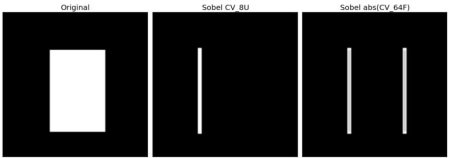# OpenCV-Python 系列 十七 | 图像梯度

### 目标

– 查找图像梯度，边缘等
– 我们将看到以下函数：cv.Sobel()，cv.Scharr()，cv.Laplacian()等

### 理论

OpenCV提供三种类型的梯度滤波器或高通滤波器，即Sobel，Scharr和Laplacian。我们将看到他们每一种。

### 1. Sobel 和 Scharr 算子

Sobel算子是高斯平滑加微分运算的联合运算，因此它更抗噪声。逆可以指定要采用的导数方向，垂直或水平（分别通过参数yorder和xorder）。逆还可以通过参数ksize指定内核的大小。如果ksize = -1，则使用3×3 Scharr滤波器，比3×3 Sobel滤波器具有更好的结果。请参阅文档以了解所使用的内核。

### 2. Laplacian 算子

$$kernel = begin{bmatrix} 0 & 1 & 0 \ 1 & -4 & 1 \ 0 & 1 & 0 end{bmatrix}$$

### 代码

import numpy as np
import cv2 as cv
from matplotlib import pyplot as plt
laplacian = cv.Laplacian(img,cv.CV_64F)
sobelx = cv.Sobel(img,cv.CV_64F,1,0,ksize=5)
sobely = cv.Sobel(img,cv.CV_64F,0,1,ksize=5)
plt.subplot(2,2,1),plt.imshow(img,cmap = 'gray')
plt.title('Original'), plt.xticks([]), plt.yticks([])
plt.subplot(2,2,2),plt.imshow(laplacian,cmap = 'gray')
plt.title('Laplacian'), plt.xticks([]), plt.yticks([])
plt.subplot(2,2,3),plt.imshow(sobelx,cmap = 'gray')
plt.title('Sobel X'), plt.xticks([]), plt.yticks([])
plt.subplot(2,2,4),plt.imshow(sobely,cmap = 'gray')
plt.title('Sobel Y'), plt.xticks([]), plt.yticks([])
plt.show()### 一个重要事项

import numpy as np
import cv2 as cv
from matplotlib import pyplot as plt
# Output dtype = cv.CV_8U
sobelx8u = cv.Sobel(img,cv.CV_8U,1,0,ksize=5)
# Output dtype = cv.CV_64F. Then take its absolute and convert to cv.CV_8U
sobelx64f = cv.Sobel(img,cv.CV_64F,1,0,ksize=5)
abs_sobel64f = np.absolute(sobelx64f)
sobel_8u = np.uint8(abs_sobel64f)
plt.subplot(1,3,1),plt.imshow(img,cmap = 'gray')
plt.title('Original'), plt.xticks([]), plt.yticks([])
plt.subplot(1,3,2),plt.imshow(sobelx8u,cmap = 'gray')
plt.title('Sobel CV_8U'), plt.xticks([]), plt.yticks([])
plt.subplot(1,3,3),plt.imshow(sobel_8u,cmap = 'gray')
plt.title('Sobel abs(CV_64F)'), plt.xticks([]), plt.yticks([])
plt.show()